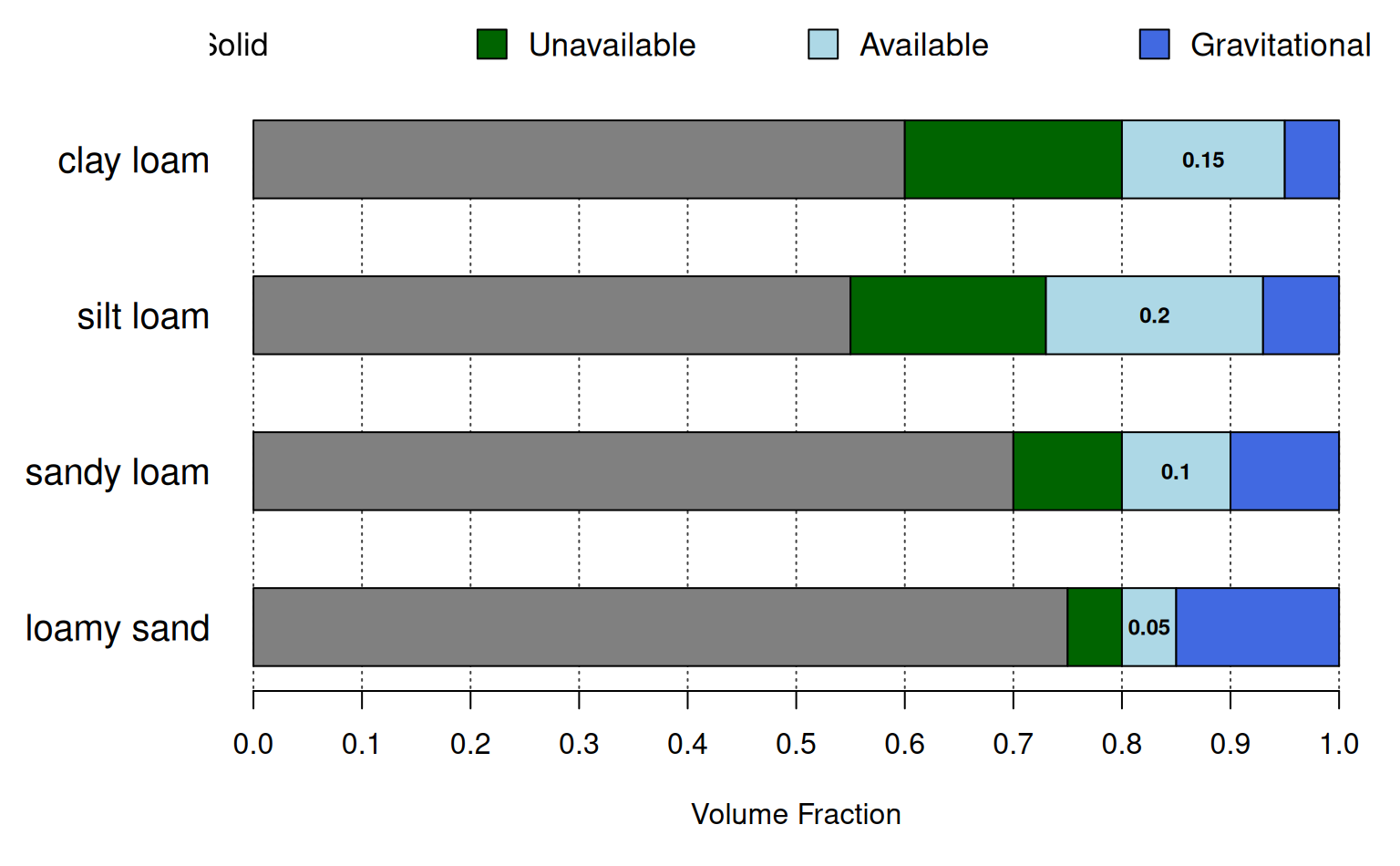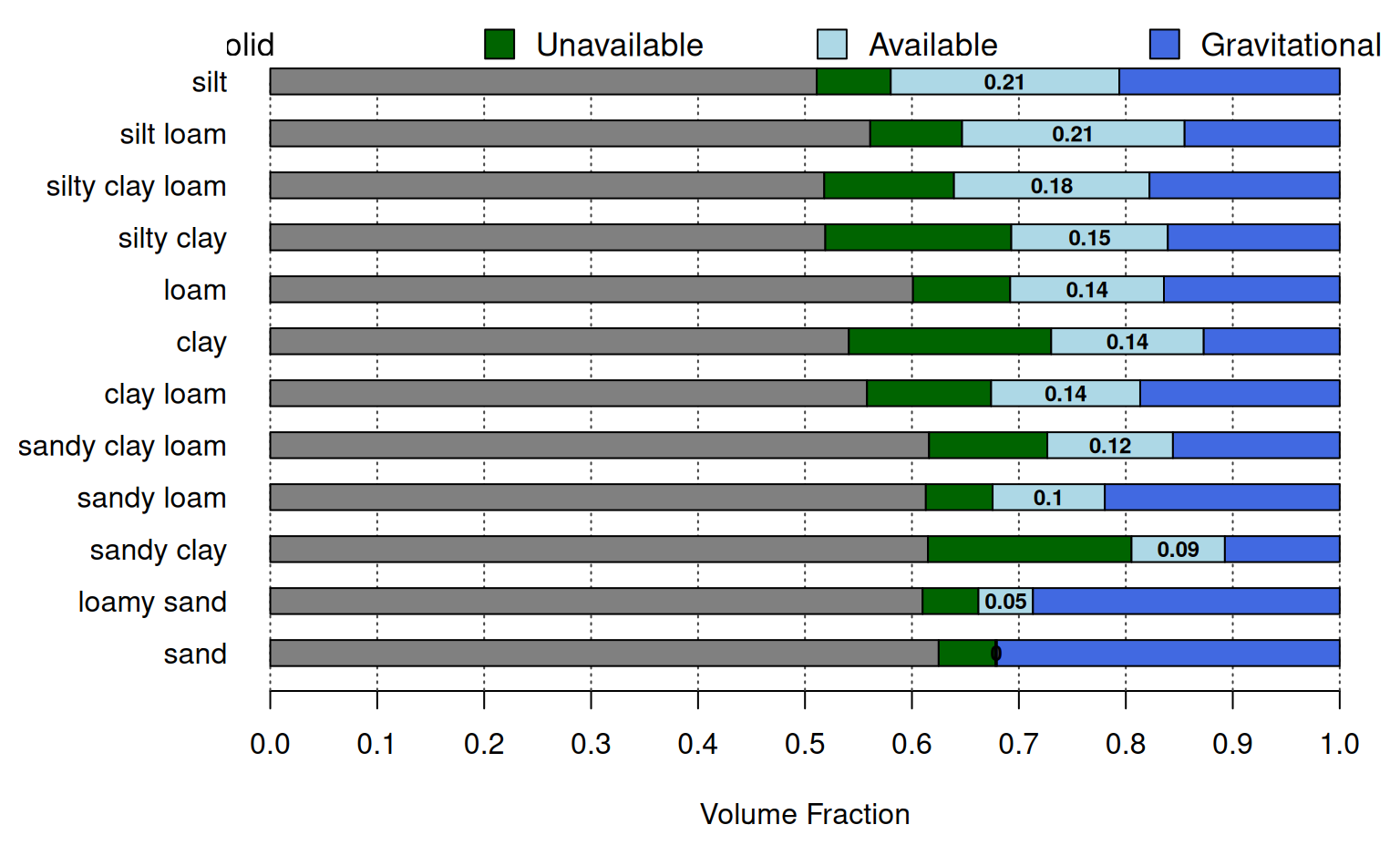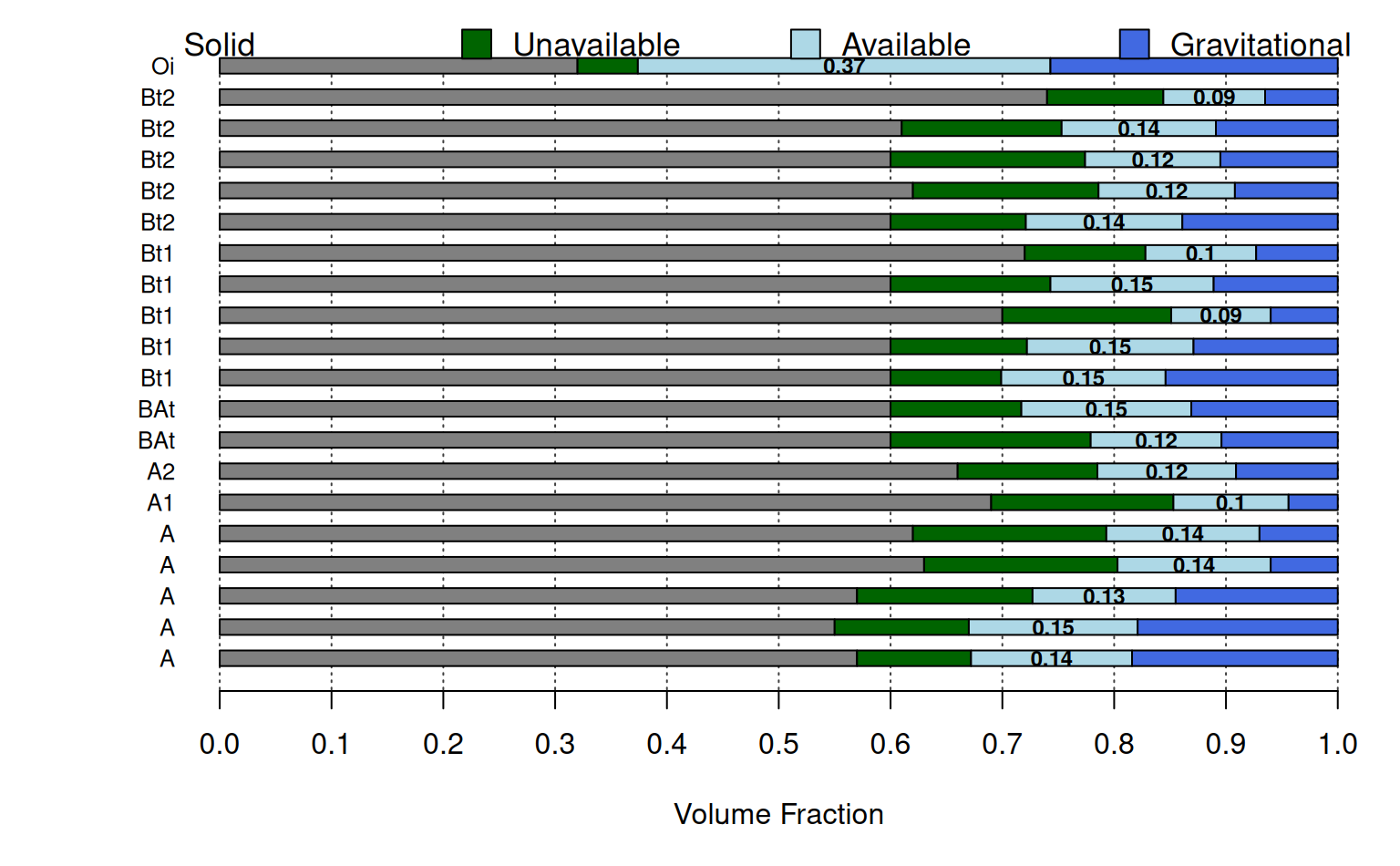Generate a simplistic diagram of the various fractions of water held within soil pore-space. Largely inspired by Figure 2 from O'Geen (2013).

plotAvailWater(
x,
width = 0.25,
cols = c(grey(0.5), "DarkGreen", "LightBlue", "RoyalBlue"),
name.cex = 0.8,
annotate = TRUE
)

## Arguments

x

a data.frame containing sample names and water retention data, see examples below

width

vertical width of each bar graph

cols

a vector of colors used to symbolize 'solid phase', 'unavailable water', 'available water', and 'gravitational water'

name.cex

character scaling of horizon names, printed on left-hand side of figure

annotate

logical, annotate AWC

## Value

nothing, function is called to generate graphical output

D.E. Beaudette

## Examples



# demonstration
s <- data.frame(
name = c('loamy sand', 'sandy loam', 'silt loam', 'clay loam'),
pwp = c(0.05, 0.1, 0.18, 0.2),
fc = c(0.1, 0.2, 0.38, 0.35),
sat = c(0.25, 0.3, 0.45, 0.4))
s$solid <- with(s, 1-sat) par(mar=c(5, 6, 0.5, 0.5)) plotAvailWater(s, name.cex=1.25)# \donttest{ if(requireNamespace("aqp")) { # demonstration using idealized AWC by soil texture data("ROSETTA.centroids", package = "aqp") # subset columns x <- ROSETTA.centroids[, c('texture', 'pwp', 'fc', 'sat', 'awc')] # adjust to expected names / additional data required by plotAvailWater names(x) <- 'name' x$solid <- with(x, 1 - sat)

# re-order based on approximate AWC
x <- x[order(x$awc), ] op <- par(no.readonly = TRUE) par(mar=c(5, 6.5, 0.5, 0.5)) plotAvailWater(x, name.cex = 1) par(op) }# use some real data from SSURGO if(requireNamespace("curl") & curl::has_internet() & require(soilDB)) { q <- "SELECT hzdept_r as hztop, hzdepb_r as hzbottom, hzname as name, wsatiated_r/100.0 as sat, wthirdbar_r/100.0 as fc, wfifteenbar_r/100.0 as pwp, awc_r as awc FROM chorizon WHERE cokey IN (SELECT cokey from component where compname = 'dunstone') AND wsatiated_r IS NOT NULL ORDER BY cokey, hzdept_r ASC;" x <- SDA_query(q) x <- unique(x) x <- x[order(x$name), ]
x\$solid <- with(x, 1-sat)